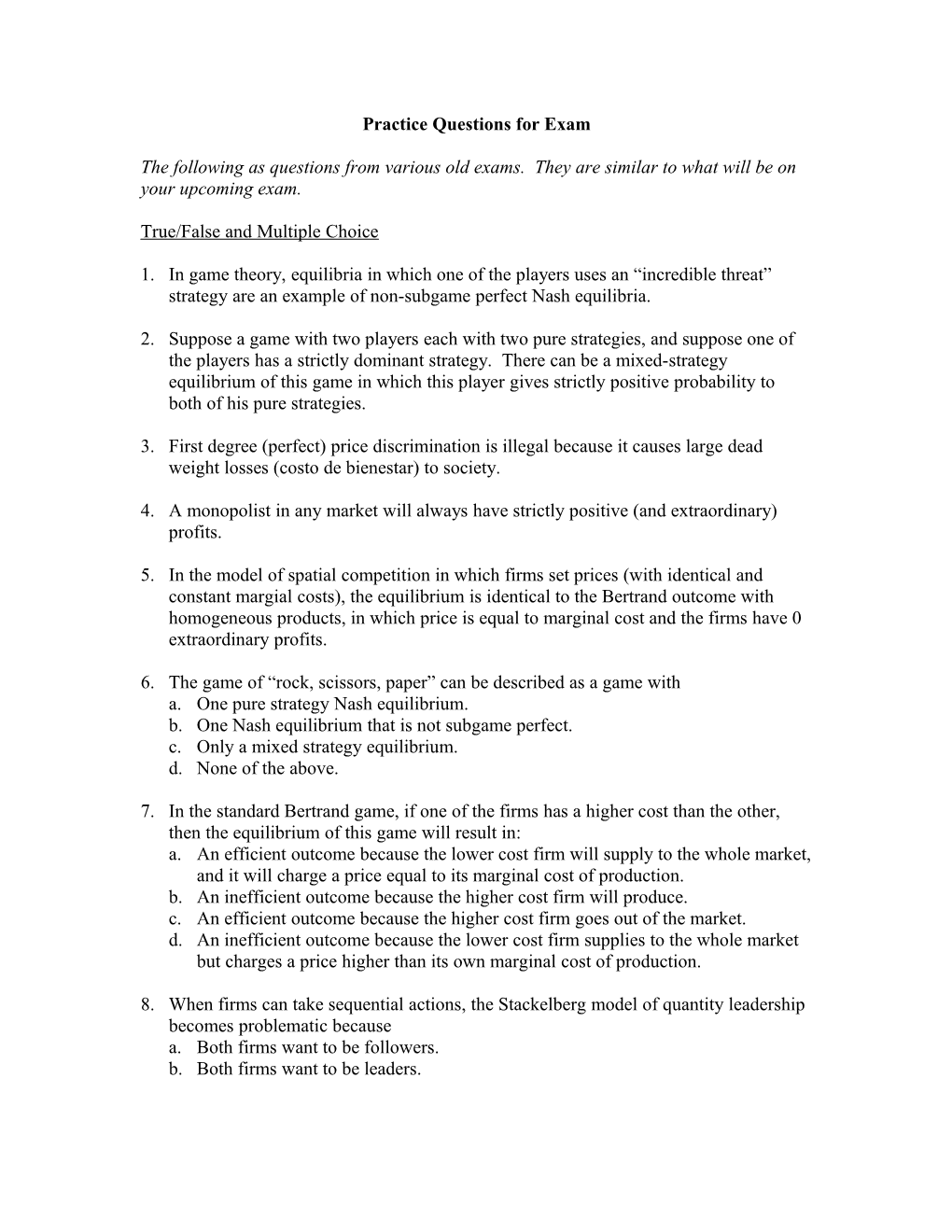# Practice Questions for ExamPractice Questions for Exam

The following as questions from various old exams. They are similar to what will be on your upcoming exam.

True/False and Multiple Choice

1. In game theory, equilibria in which one of the players uses an “incredible threat” strategy are an example of non-subgame perfect Nash equilibria.
1. Suppose a game with two players each with two pure strategies, and suppose one of the players has a strictly dominant strategy. There can be a mixed-strategy equilibrium of this game in which this player gives strictly positive probability to both of his pure strategies.
1. First degree (perfect) price discrimination is illegal because it causes large dead weight losses (costo de bienestar) to society.
1. A monopolist in any market will always have strictly positive (and extraordinary) profits.
1. In the model of spatial competition in which firms set prices (with identical and constant margial costs), the equilibrium is identical to the Bertrand outcome with homogeneous products, in which price is equal to marginal cost and the firms have 0 extraordinary profits.
1. The game of “rock, scissors, paper” can be described as a game with
1. One pure strategy Nash equilibrium.
2. One Nash equilibrium that is not subgame perfect.
3. Only a mixed strategy equilibrium.
4. None of the above.
1. In the standard Bertrand game, if one of the firms has a higher cost than the other, then the equilibrium of this game will result in:
1. An efficient outcome because the lower cost firm will supply to the whole market, and it will charge a price equal to its marginal cost of production.
2. An inefficient outcome because the higher cost firm will produce.
3. An efficient outcome because the higher cost firm goes out of the market.
4. An inefficient outcome because the lower cost firm supplies to the whole market but charges a price higher than its own marginal cost of production.
1. When firms can take sequential actions, the Stackelberg model of quantity leadership becomes problematic because
1. Both firms want to be followers.
2. Both firms want to be leaders.
3. Each firm plays its best response function, taking as given the strategy of the other firm.
4. None of the above.

Problems

1. Consider the following version of a “Battle of the Sexes” game

Husband / Wife
Boxing fight / Opera
Boxing fight / 3,1 / -1,-1
Opera / -1,-1 / 1,3
1. Does this game have a Nash equilibrium in pure strategies? Is this equilibrium unique?
2. Find a Nash equilibrium in mixed strategies. Show your work carefully.
1. Consider the following majority voting game. There are three citizens in country K. Their names are A, B and C. There are three possible alternatives to vote for: X, Y, and Z. The alternative that gets the majority of votes wins. The payoffs of the players depend exclusively on which alternative is chosen through voting. Their payoffs are as follows:

UA(X)=UB(Y)=UC(Z)=2; UA(Y)=UB(Z)=UC(X)=1; UA(Z)=UB(X)=UC(Y)=0.

(You cannot draw a box diagram for this game, since there are more than 2 players.) Use the definition of Nash equilibrium to determine whether there are any Nash equilibria in pure strategies for this game. Show your work carefully. (You may find more than one Nash equilibrium).

1. Suppose a monopolist can produce any level of output it wishes at a constant marginal=average cost of \$5 per unit. Assume this monopolist sells its product in two different markets that are separated by a great distance. The demand curves in these markets are given by Q1=55-P1 and Q2=70-P2.
1. If the monopolist can maintain perfect separation between these markets, what price will he charge in each market, and what quantity will be produce in each market? What are the monopolist’s total profits?
2. Suppose now that it costs any buyer \$5 per unit to transport goods he has bought in one market and sell them in the other market. What prices would the monopolist charge now, and what would be the quantities sold in each market and the profits of the monopolist?
3. Now suppose that it costs nothing to transport the goods from one market to the other. Can the monopolist now discriminate in prices? Why or why not? Find the price or prices the monopolist will charge, as well as his quantities sold and his profits.